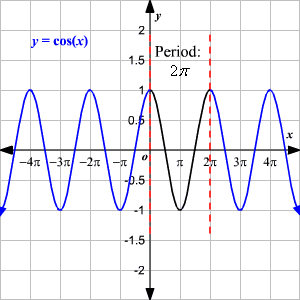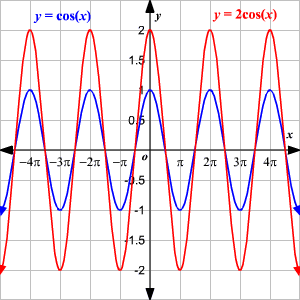# Graphing Cosine Function

The trigonometric ratios can also be considered as functions of a variable which is the measure of an angle. This angle measure can either be given in degrees or radians . Here, we will use radians.

The graph of a cosine function $y=\mathrm{cos}\left(x\right)$ is looks like this:### Properties of the Cosine Function, $y=\mathrm{cos}\left(x\right)$ .

Domain : $\left(-\infty ,\infty \right)$

Range : $\left[-1,1\right]$ or $-1\le y\le 1$

$y$ -intercept : $\left(0,1\right)$

$x$ -intercept : $\left(\frac{n\pi }{2},0\right)$ , where $n$ is an integer.

Period: $2\pi$

Continuity: continuous on $\left(-\infty ,\infty \right)$

Symmetry: $y$ -axis (even function)

The maximum value of $y=\mathrm{cos}\left(x\right)$ occurs when $x=2n\pi$ , where $n$ is an integer.

The minimum value of $y=\mathrm{cos}\left(x\right)$ occurs when $x=\pi +2n\pi$ , where $n$ is an integer.

### Amplitude and Period a Cosine Function

The amplitude of the graph of $y=a\mathrm{cos}\left(bx\right)$ is the amount by which it varies above and below the $x$ -axis.

Amplitude = | $a$ |

The period of a cosine function is the length of the shortest interval on the $x$ -axis over which the graph repeats.

Period = $\frac{2\pi }{|b|}$

Example:

Sketch the graphs of $y=\mathrm{cos}\left(x\right)$ and $y=2\mathrm{cos}\left(x\right)$ . Compare the graphs.

For the function $y=2\mathrm{cos}\left(x\right)$ , the graph has an amplitude $2$ . Since $b=1$ , the graph has a period of $2\pi$ . Thus, it cycles once from $0$ to $2\pi$ with one maximum of $2$ , and one minimum of $-2$ .Observe the graphs of $y=\mathrm{cos}\left(x\right)$ and $y=2\mathrm{cos}\left(x\right)$ . Each has the same $x$ -intercepts, but $y=2\mathrm{cos}\left(x\right)$ has an amplitude that is twice the amplitude of $y=\mathrm{cos}\left(x\right)$ .

Also see Trigonometric Functions .